图解：有向环、拓扑排序与 Kosaraju 算法﻿﻿

﻿

﻿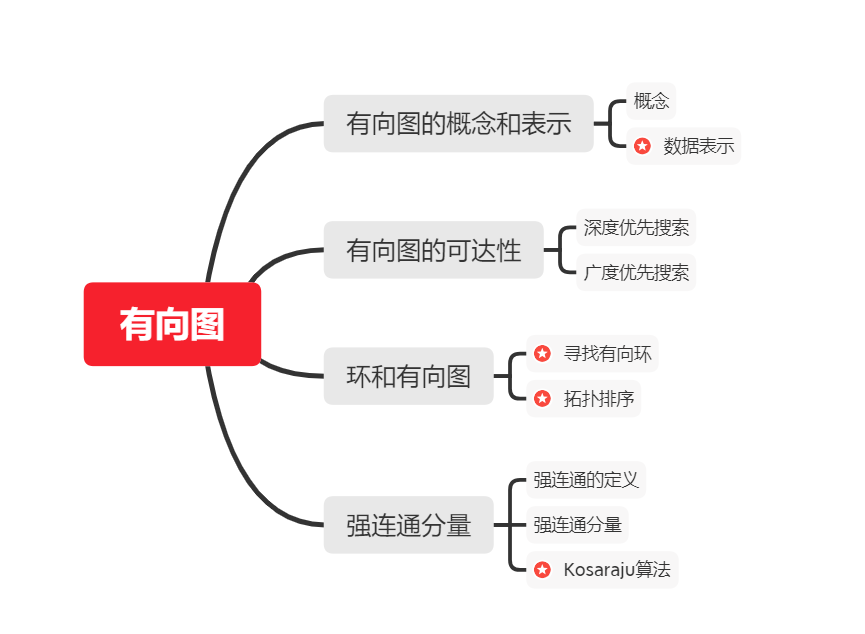﻿

1.有向图的概念和表示

﻿

概念

﻿

>一幅有方向的图（或有向图）是由一组顶点和一组有方向的边组成的，每条有方向的边都连接着一对有序的顶点。

﻿

﻿

数据表示

﻿

﻿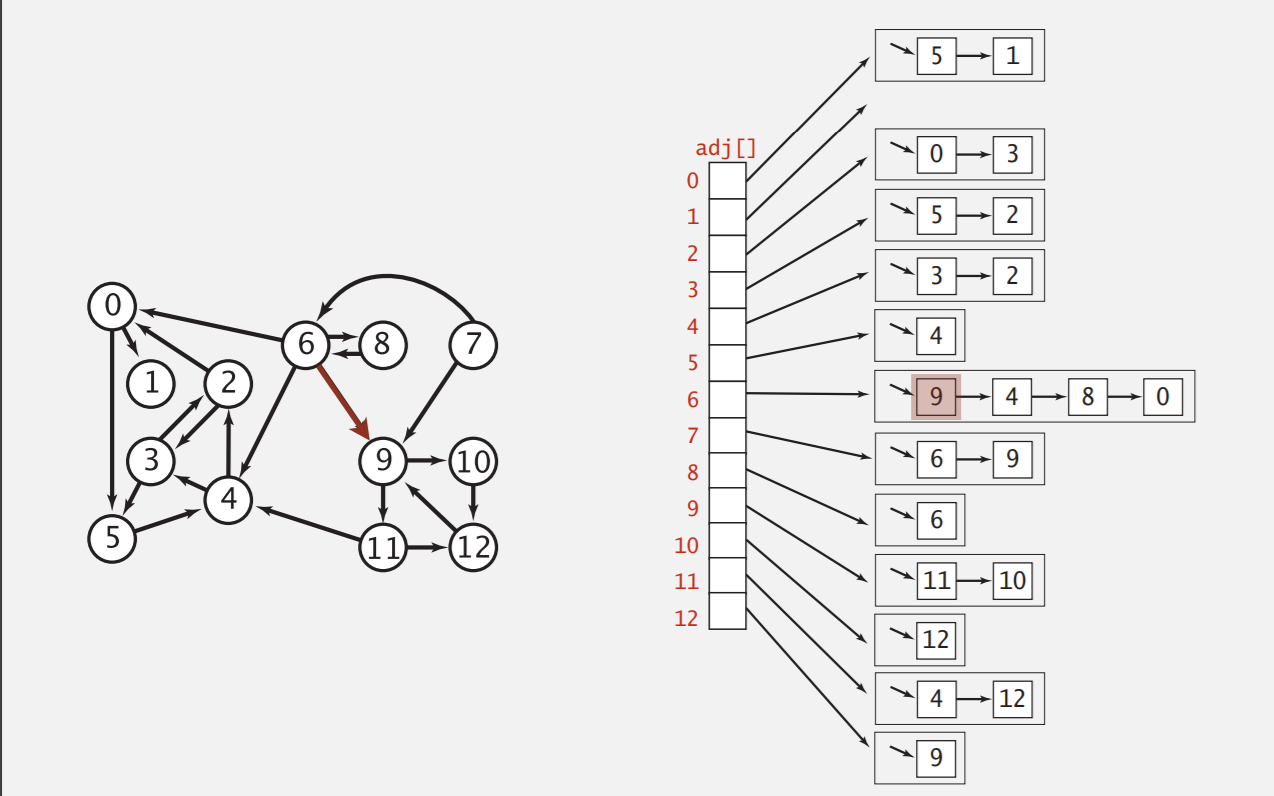﻿

package Graph.Digraph;
public class Digraph{
private final int V;//顶点数目
private int E;//边的数目
public Digraph(int V){
//创建邻接表
//将所有链表初始化为空
this.V=V;this.E=0;
for(int v=0;v<V;++v){
}
}
public int V(){ return V;}//获取顶点数目
public int E(){ return E;}//获取边的数目
//注意，只有这里与无向图不同
E++;
}
}
//获取有向图的取反
public Digraph reverse(){
Digraph R=new Digraph(V);
for(int v=0;v<V;v++){
}
return R;
}
}

﻿

﻿

﻿

3.环和有向无环图

﻿

﻿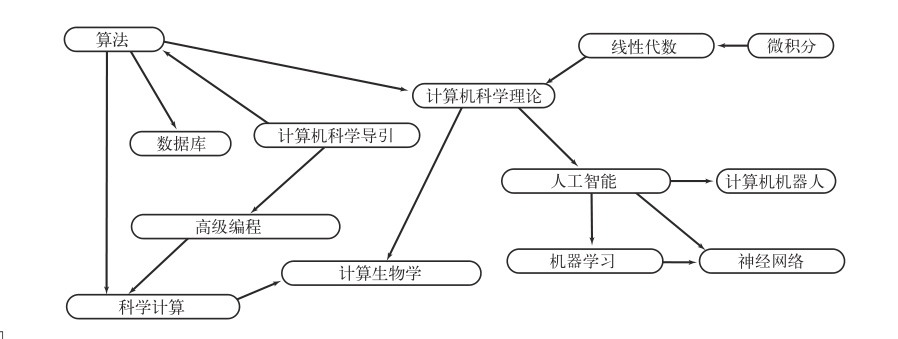﻿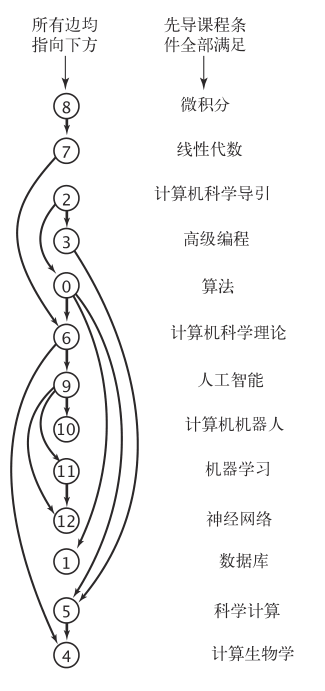﻿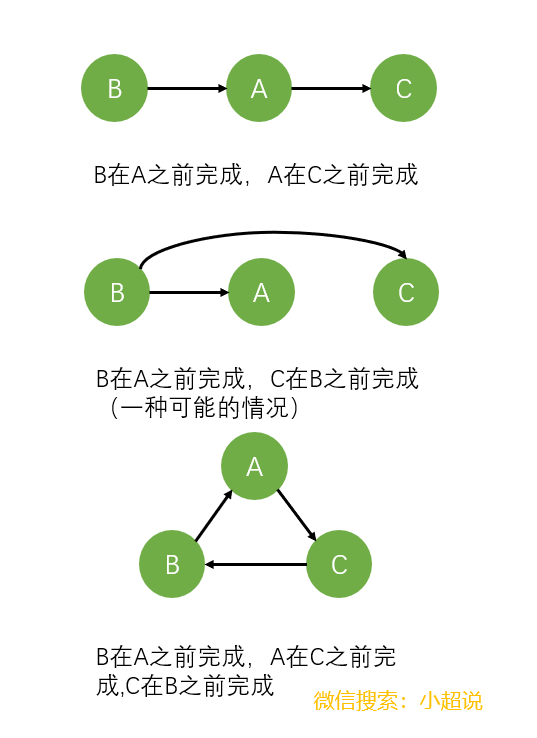﻿

﻿

• 如何检测有向环（只检查存在性，不考虑有多少个）

• 对于一个不存在有向环的有向图，如何排序找到解决方案（任务调度问题）

﻿

1.寻找有向环

﻿

﻿

• 基本的dfs算法

• 新增一个onStack[]数组用来显式地记录栈上的顶点（即一个顶点是否在栈上）

﻿

﻿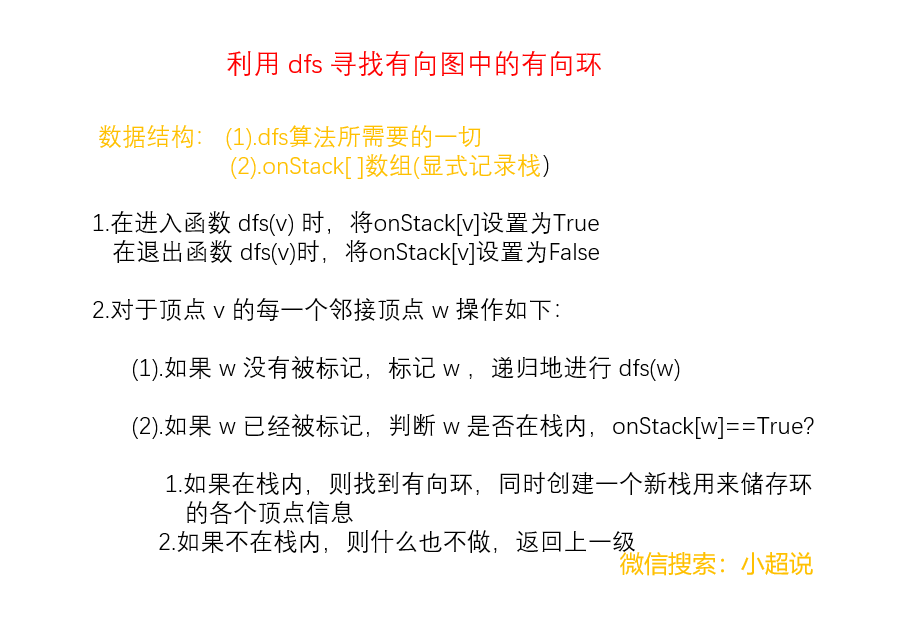﻿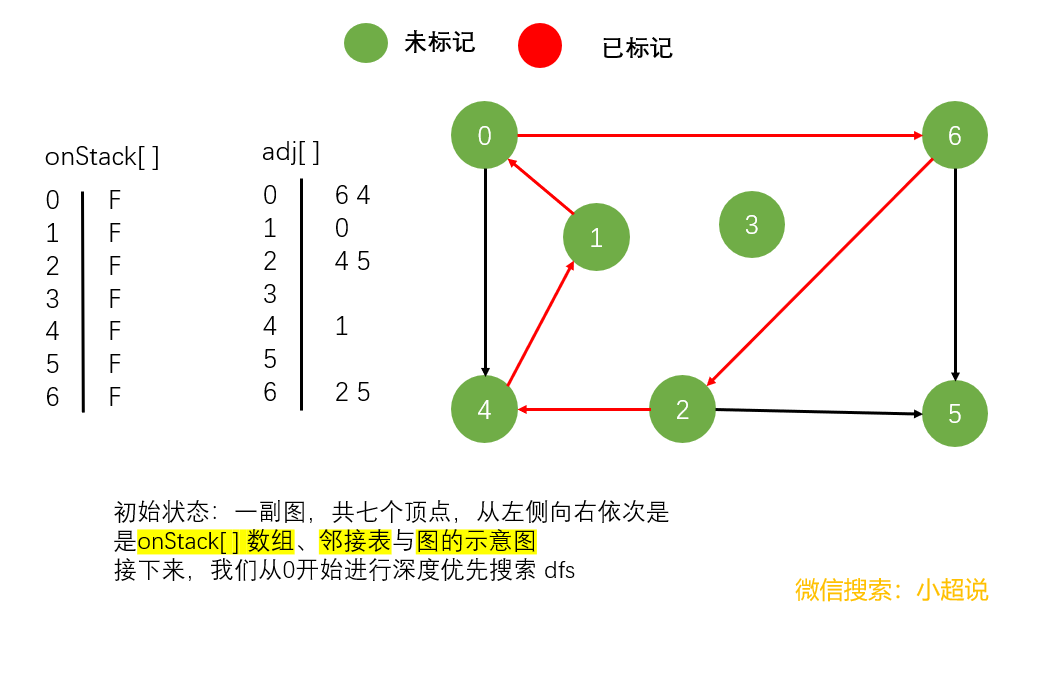﻿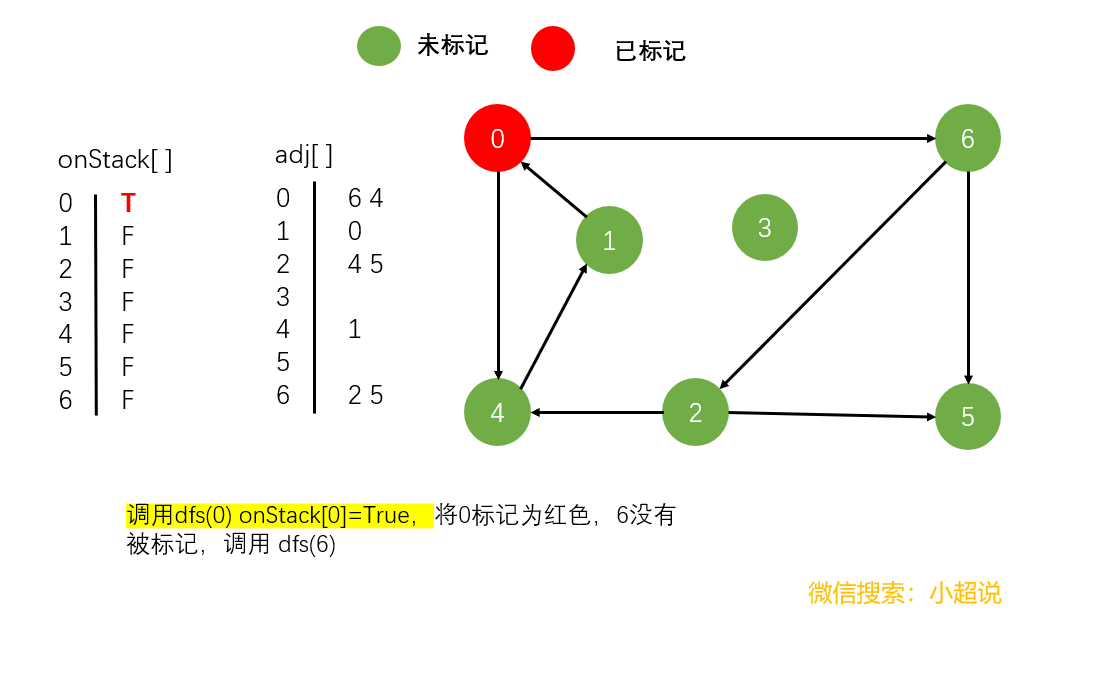﻿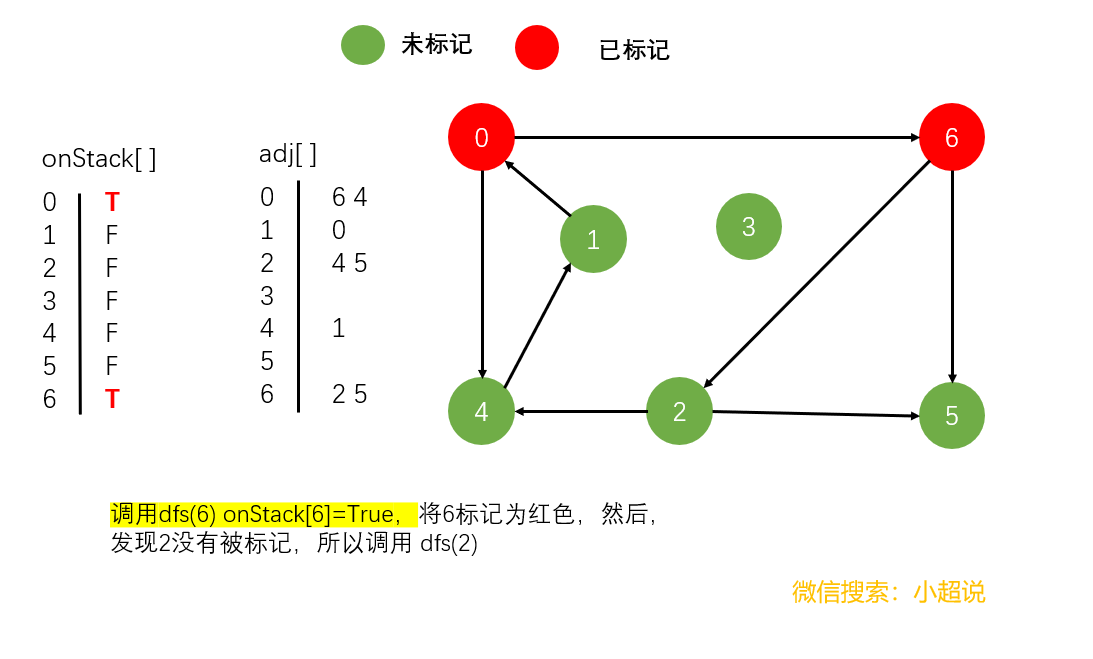﻿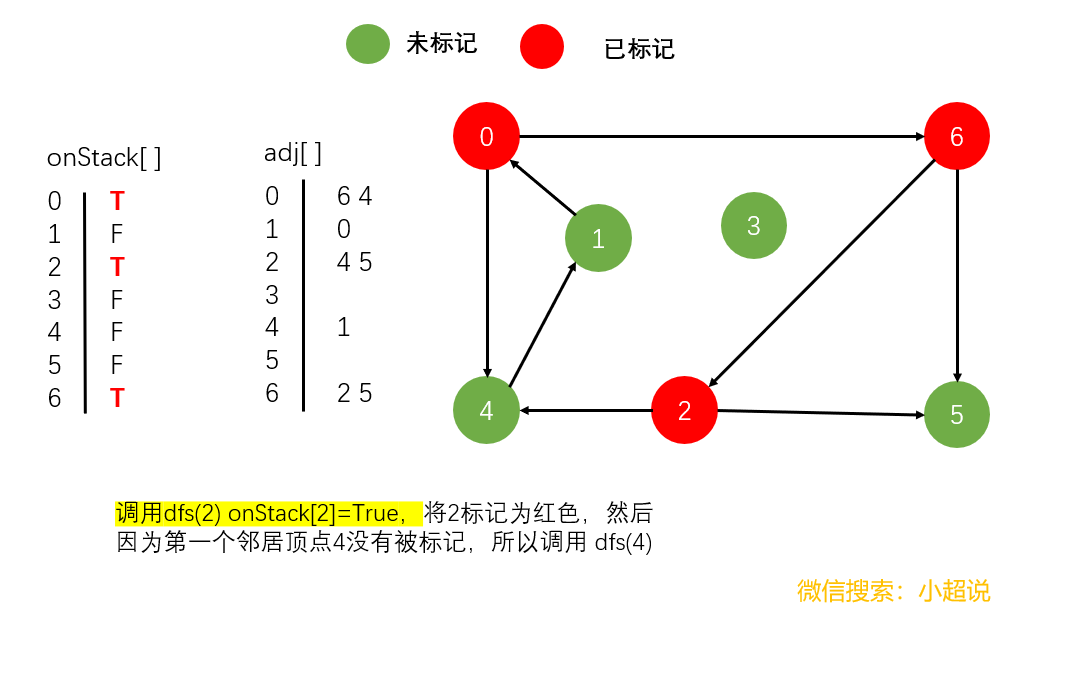﻿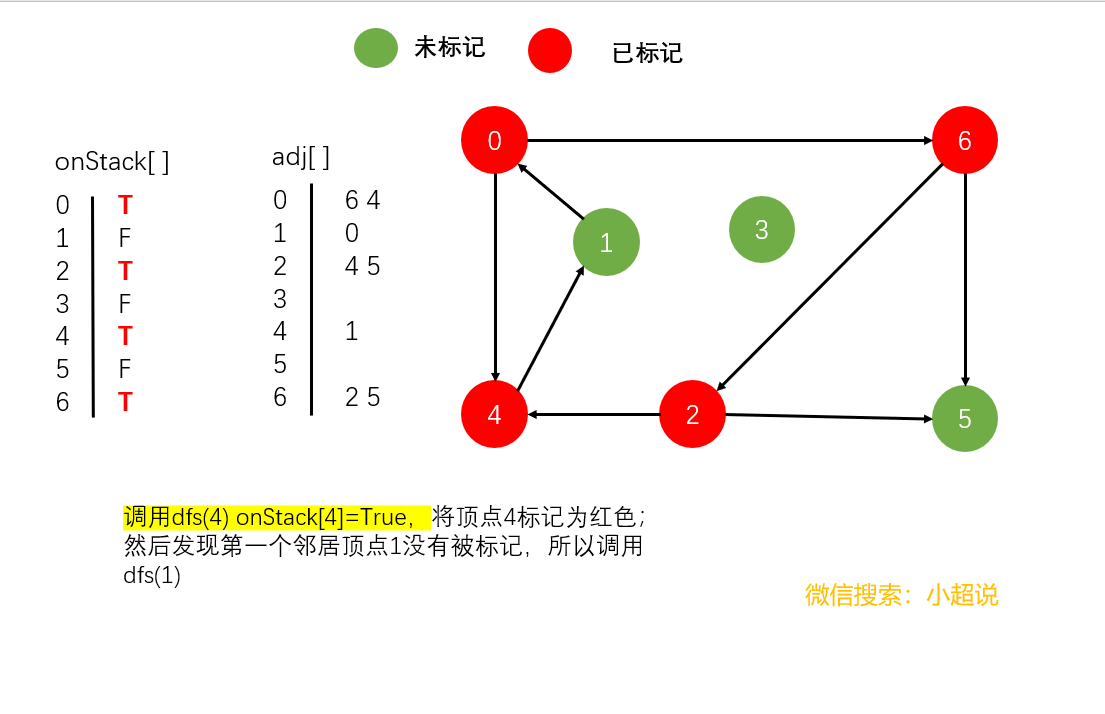﻿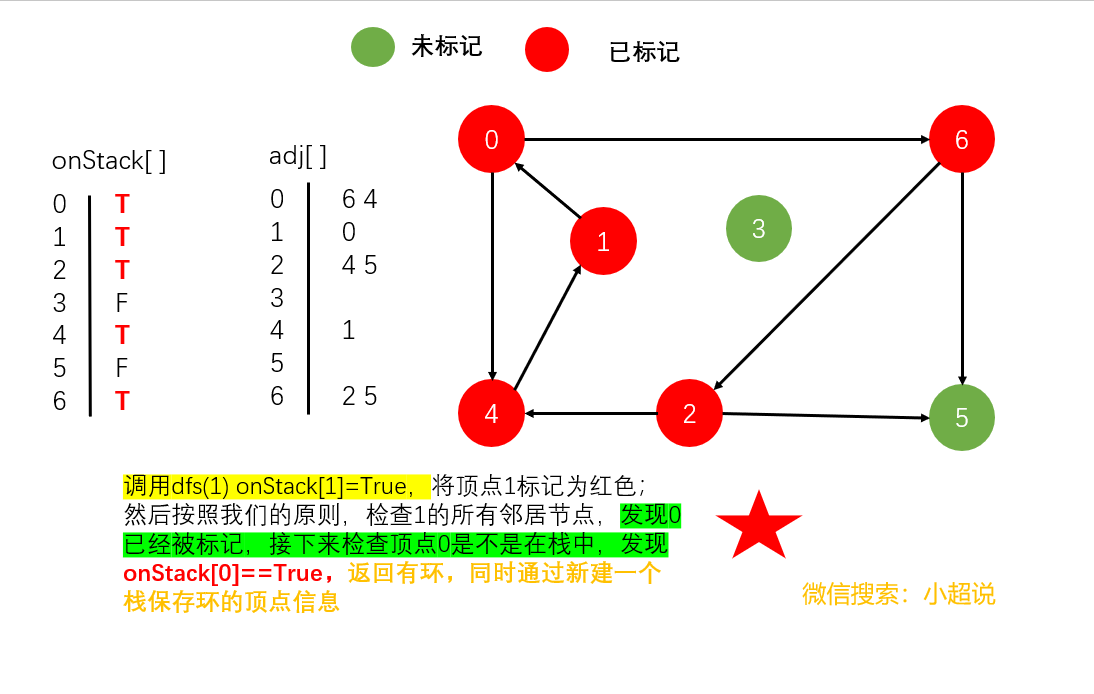﻿

﻿

package Graph.Digraph;
import java.util.Stack;
public class DirectedCycle {
private boolean [] marked;
private int [] edgeTo;
private Stack<Integer> cycle;//有向环中的所有顶点（如果存在）
private boolean[] onStack; //递归调用的栈上的所有顶点
public DirectedCycle(Digraph G){
onStack=new boolean[G.V()];
edgeTo=new int[G.V()];
marked=new boolean[G.V()];
for(int v=0;v<G.V();v++){
if(!marked[v]) dfs(G,v);
}
}
private void dfs(Digraph G,int v){
onStack[v]=true;//进入dfs时，顶点v入栈
marked[v]=true;
if(this.hasCycle()) return;
else if(!marked[w]){
edgeTo[w]=v;dfs(G,w);
}
else if(onStack[w]){
//重点
cycle=new Stack<Integer>();
for(int x=v;x!=w;x=edgeTo[x])
cycle.push(x);
cycle.push(w);
cycle.push(v);
}
}
//退出dfs时，将顶点v出栈
onStack[v]=false;
}
public boolean hasCycle(){
return cycle!=null;
}
public Iterable<Integer> cycle(){
return cycle;
}
}

﻿

edgeTo[] 中的链接得到。

﻿

dfs(G,v) 时将 onStack[v] 设为 True，在调用结束时将其设为 false）。DirectedCycle 同时也

﻿

2.拓扑排序

﻿

﻿

>拓扑排序的定义：给定一幅有向图，将所有的顶点排序，使得所有的有向边均从排在前面的元素指向排在后面的元素（或者说明无法做到这一点）

﻿

﻿﻿﻿

﻿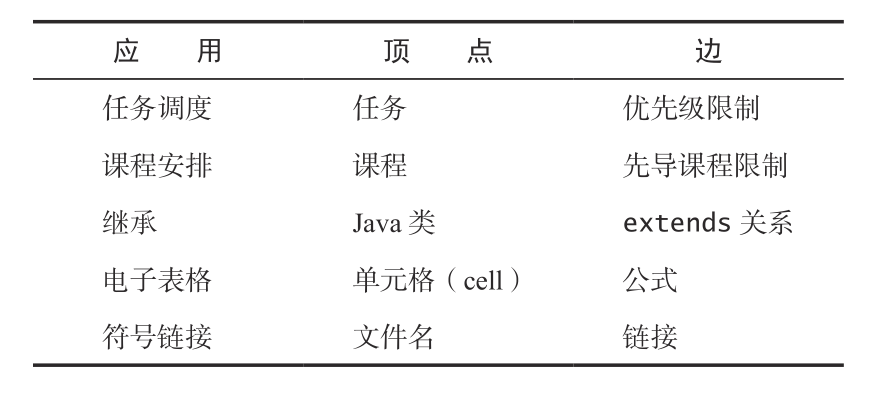﻿

﻿

﻿

• dfs(w)已经被调用过且已经返回了（此时w已经被标记）

• dfs(w)已经被调用过且还没有返回（仔细想想这种情况，这是不可能存在的）

• dfs(w)还没有被调用（w还没有被标记），此时情况并不复杂，接下来会调用dfs(w)，然后返回dfs(w)，然后调用dfs(v)

﻿

﻿

﻿

• 前序：在递归调用之前将顶点加入队列

• 后序：在递归调用之后将顶点加入队列

• 逆后序：在递归调用之后将顶点压入栈

﻿

﻿

package Graph.Digraph;
import java.util.ArrayList;
import java.util.Queue;
import java.util.Stack;
public class DepthFirstOrder {
private boolean [] marked;
private Queue<Integer> pre;//所有顶点的前序排列
private Queue<Integer> post;//所有顶点的后序排列
private Stack<Integer> reversePost;//所有顶点的逆后序排列
public DepthFirstOrder(Digraph G){
reversePost = new Stack<>();
marked=new boolean[G.V()];
for(int v=0;v<G.V();v++){
if(!marked[v]) dfs(G,v);
}
}
private void dfs(Digraph G,int v){
pre.offer(v);
marked[v]=true;
if(!marked[w])
dfs(G, w);
post.offer(v);
reversePost.push(v);
}
//这里可以不用管
public Iterable<Integer> pre()
{ return pre; }
public Iterable<Integer> post()
{ return post; }
public Iterable<Integer> reversePost()
{ return reversePost; }
}

﻿

﻿

package Graph.Digraph;
public class Topological {
private Iterable<Integer> order;//顶点的拓扑顺序
public Topological(Digraph G){
//判断给定的图G是否有环
DirectedCycle cyclefinder=new DirectedCycle(G);
if(!cyclefinder.hasCycle()){
DepthFirstOrder dfs=new DepthFirstOrder(G);
order = dfs.reversePost();
}
}
public Iterable<Integer> order(){
return order;
}
//判断图G是不是有向无环图
public boolean isDAG(){
return order!=null;
}
}

﻿

﻿

4.强连通分量

﻿

1.强连通的定义

﻿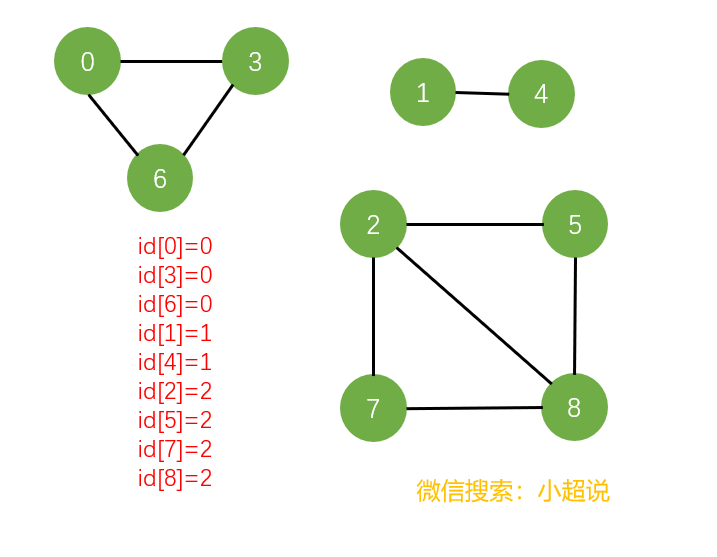﻿

﻿

﻿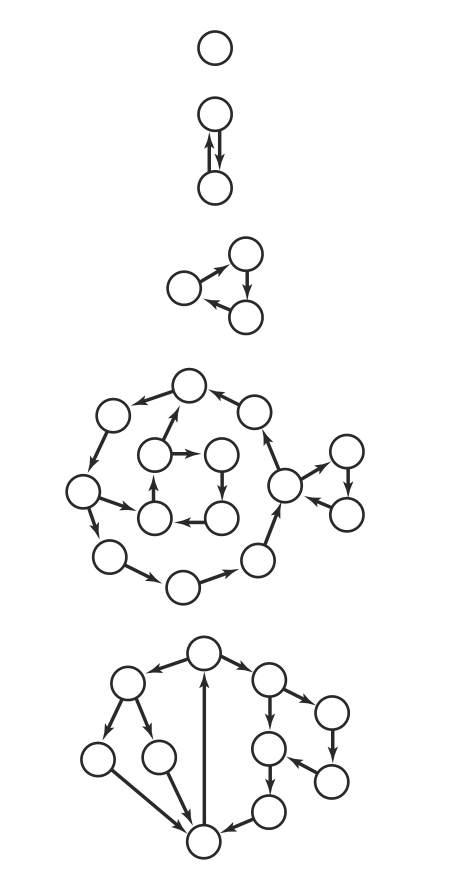﻿

2.强连通分量

﻿

• 自反性：任意顶点 v 和自己都是强连通的

• 对称性：如果 v 和 w 是强连通的，那么 w 和 v 也是强连通的

• 传递性：如果 v 和 w 是强连通的且 w 和 x 也是强连通的，那

﻿

﻿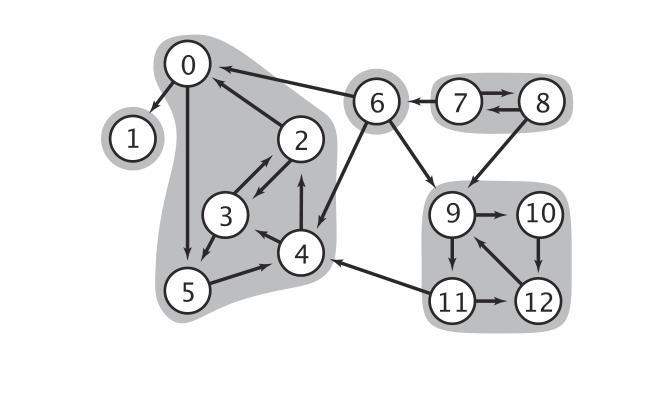﻿

﻿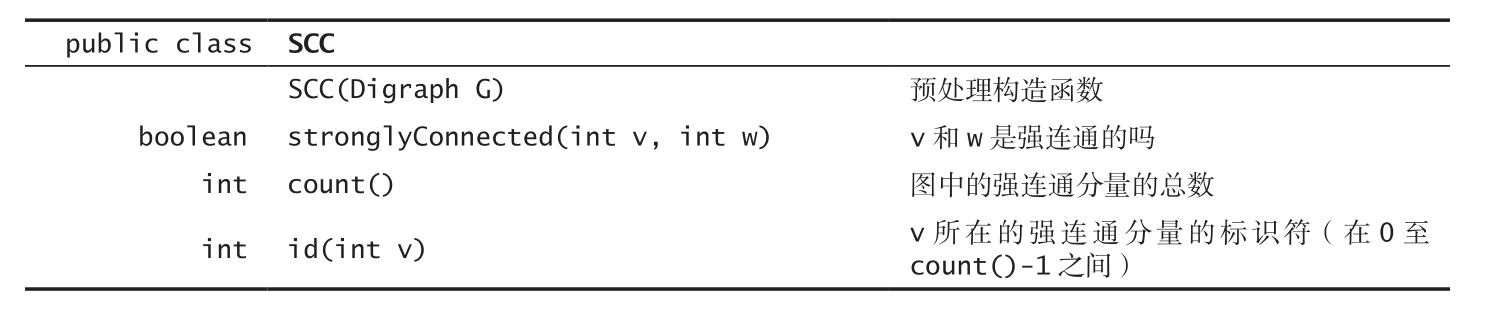﻿

3.Kosaraju算法

﻿

Kosaraju算法就是一种经典的解决强连通性问题的算法，它实现很简单，但是不好理解why，希望你打起精神，我希望我能够把它讲明白（也只是希望，我会尽量，如果不清楚的话，强烈建议结合算法4一起食用）

﻿

﻿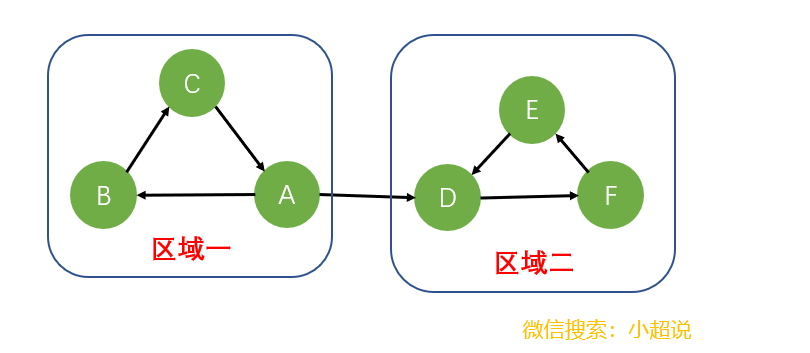﻿

﻿

﻿

﻿

﻿

﻿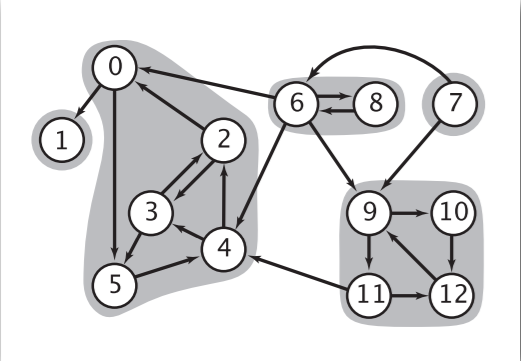﻿

ps:想一想为什么不能存在环呢？因为前提我们把所有的强连通分量看成了一个个顶点，如果顶点A顶点B之间存在环，那AB就会构成一个更大的强连通分量！它们本应属于一个顶点！

﻿

﻿

﻿

﻿

﻿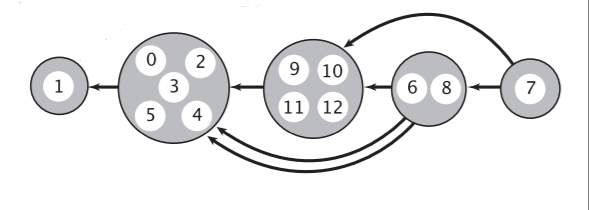﻿

﻿

﻿

﻿

﻿

• 1.在给定的一幅有向图 G 中，使用 DepthFirstOrder 来计算它的反向图 GR 的逆后序排列。

• 2.在 G 中进行标准的深度优先搜索，但是要按照刚才计算得到的顺序而非标准的顺序来访问

﻿

﻿

package Graph.Digraph;
public class KosarajuSCC
{
private boolean[] marked; // 已访问过的顶点
private int[] id; // 强连通分量的标识符
private int count; // 强连通分量的数量
public KosarajuSCC(Digraph G)
{
marked = new boolean[G.V()];
id = new int[G.V()];
DepthFirstOrder order = new DepthFirstOrder(G.reverse()); //重点
for (int s : order.reversePost()) //重点
if (!marked[s])
{ dfs(G, s); count++; }
}
private void dfs(Digraph G, int v)
{
marked[v] = true;
id[v] = count;
if (!marked[w])
dfs(G, w);
}
public boolean stronglyConnected(int v, int w)
{ return id[v] == id[w]; }
public int id(int v)
{ return id[v]; }
public int count()
{ return count;}
}

﻿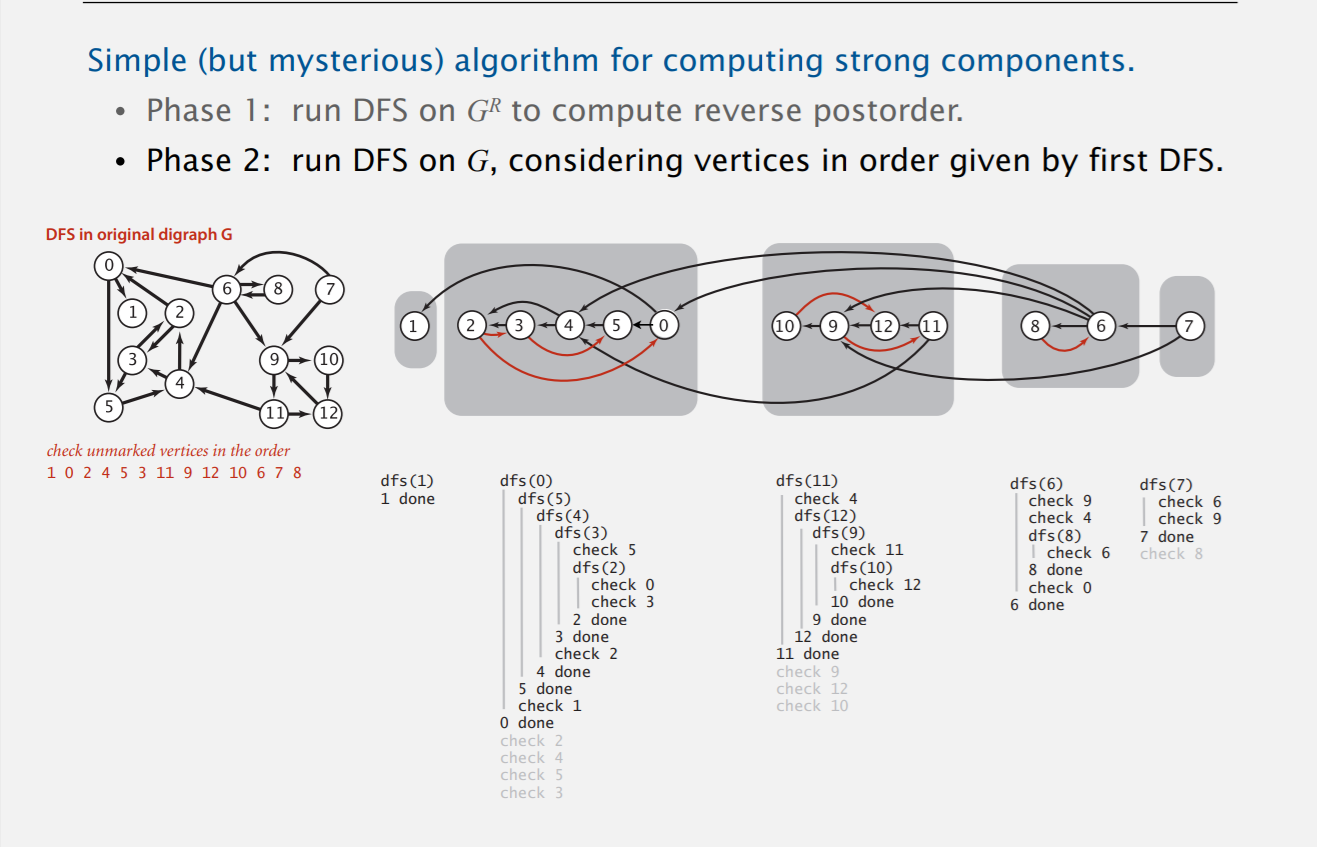﻿

• 给定的两个顶点的连通性（上文代码stronglyConnected)

• 该图中有多少个强连通分量（上文代码count)

﻿

后记

﻿

﻿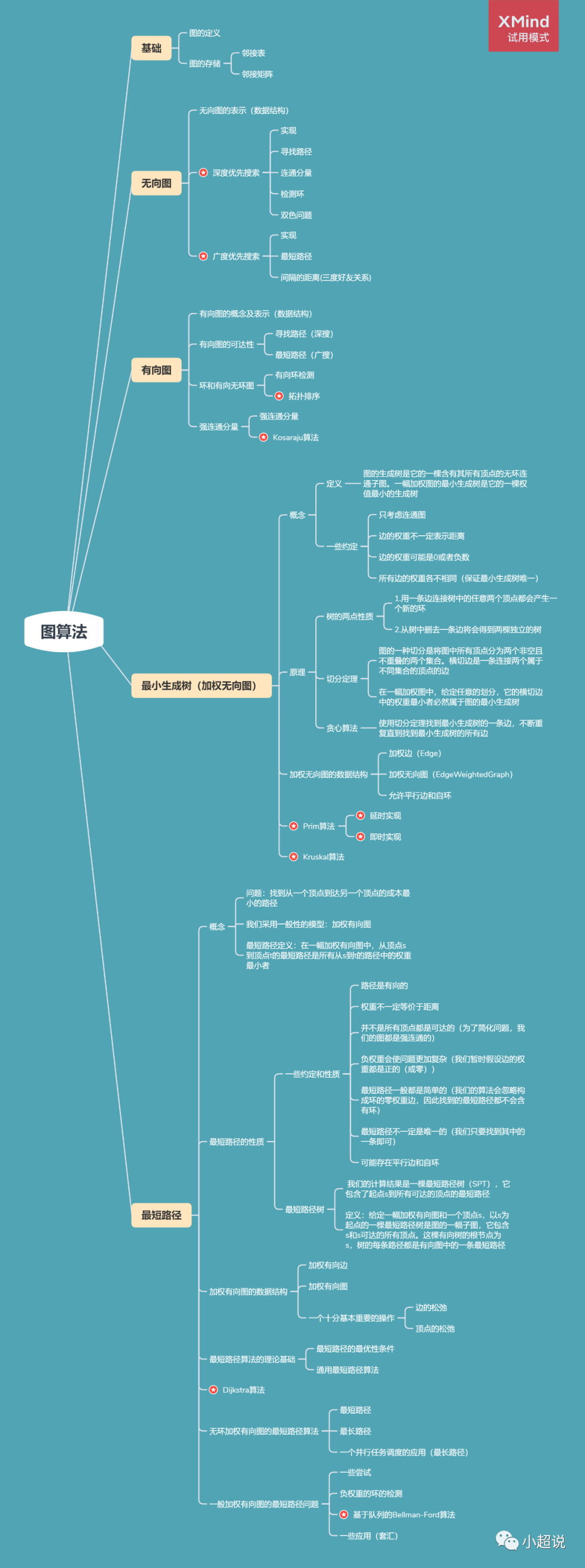﻿

﻿

﻿

﻿﻿评论